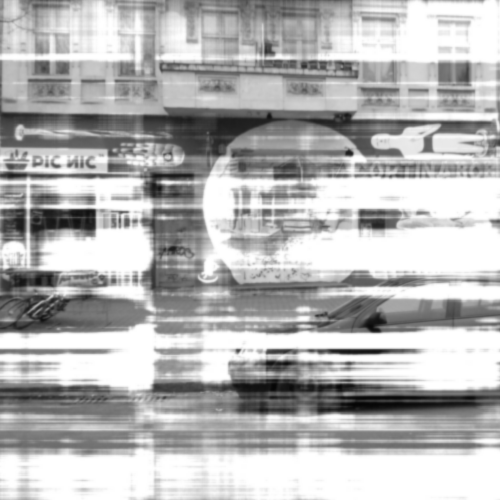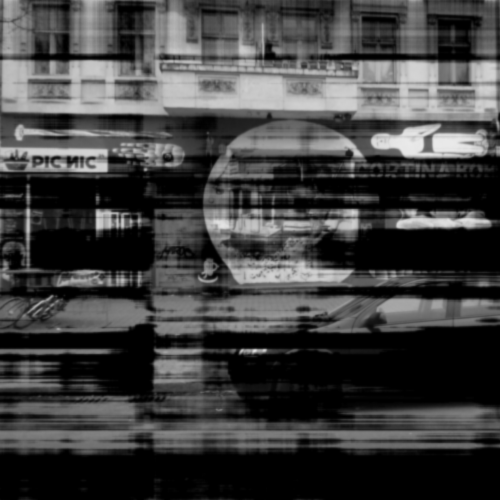This evening I didn’t feel like doing homework, so I decided to implement the databending effect (from my MFA show) in Processing (with a view to a future Android application). This databending effect stems from an error in precision in [jit.la.inverse] in Max, resulting in error matrices which can be added or subtracted from images to simulate the databent effect.

So I took png versions of these error matrices, and implemented a simple Processing sketch which one can choose between different ‘errors’ (up and DOWN keyboard arrows), the effect’s intensity (left-to-right mouseX), and wether the effect should be added or subtracted (mouseY).

``````PImage img, db;
int intensity = 5;
int imageNumber = 0;
int snapshotCount = 0;
boolean subtractiveFX = true;

void setup()
{
//set screen size
size(500, 500);
}

void draw()
{
intensity = (int) (10.0 * (mouseX/(width*1.0)));
subtractiveFX = ( mouseY < height/2 ? true : false );

//convert img to black and white
for(int i = 0; i < width*height; i++)
{
color c = img.pixels[i];
int gray = (int)( (red(c) + green(c) + blue(c))/3);
img.pixels[i] = color(gray, gray, gray);
}

for(int i = 0; i < width*height; i++)
{
float org = red(img.pixels[i]);
float fx = red(db.pixels[i]);
int result;
if( subtractiveFX )
{
result = (int) (org - intensity*fx);
result = ( result < 0 ? 0 : result );
}
else
{
result = (int) (org + intensity*fx);
result = ( result > 255 ? 255 : result );
}
img.pixels[i] = color(result, result, result);
}

//display on screen and add a small blur
image(img, 0, 0);
filter(BLUR, 1);
}

void keyPressed()
{
switch( keyCode )
{
case 38: //UP - change to next db image
{
imageNumber = ( imageNumber < 9 ? imageNumber+1 : 9 );
break;
}
case 40: //DOWN - change to previous db image
{
imageNumber = ( imageNumber > 0 ? imageNumber-1 : 0 );
break;
}
}

if( key == 's' || key == 'S' ) //take a snapshot of the current image
{
saveFrame("s"+snapshotCount+".png");
snapshotCount++;
}
}
``````

So, an image I uploaded to instagram this morningcan be effected as followsorTomorrow I am going to implement this for iOS and experiment more. In the meantime, the source code and error images can be found here.